## 整数的展开

2011-03-14 00:33  Anders Cui  阅读(2605)  评论(2编辑  收藏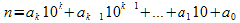（公式1）

nb进制展开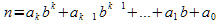（公式2）

private static readonly string digitTable = "0123456789abcdef";private static readonly int maxBase = digitTable.Length;/// <summary>/// Base b expansion./// </summary>/// <param name="n">n >= 0</param>/// <param name="b">b in [2, 16]</param>/// <returns></returns>public static string Expansion(int n, int b){    Stack<string> remainders = new Stack<string>();    do    {        remainders.Push((n % b).ToString());        n /= b;    } while (n > 0);    return string.Join(string.Empty, remainders.ToArray());}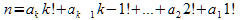，其中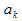为整数，且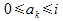，i=1,2,...,n。